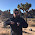## Wednesday, January 24, 2018

### Varying $\langle k \rangle$

As I mention in my (newly revised) recent paper, we can use the ensemble approach to arrive at an almost identical information equilibrium condition for an ensemble of markets:

$$\frac{d \langle A \rangle}{dB} = \langle k \rangle \; \frac{\langle A \rangle}{B}$$

If $\langle k \rangle$ is slowly varying enough to treat as a constant $\bar{k}$, then we obtain the same solutions we have for a single market. But what if $\langle k \rangle$ has a small dependence on $B$

$$\langle k \rangle \approx \bar{k} + \beta \frac{B}{B_{0}}$$

Where $\beta \ll 1$? The result is actually pretty straightforward (the differential equation is still exactly solvable )

$$\frac{\langle A \rangle }{A_{0}} \approx \left( \frac{B}{B_{0}} \right)^{\bar{k}} \left( 1 + \beta \frac{B}{B_{0}}\right)$$

...

Footnotes:

 The exact solution is

$$A(B) = A_{0} \exp \left( \beta \frac{B}{B_{0}} + \bar{k} \log \frac{B}{B_{0}} \right)$$
but since $\beta$ is small, we can expand the exponential and rewrite it in the more familiar form showing the $\beta$ term as a perturbation.

1.Am I correct in thinking that term 'bracket A bracket' is a collection that includes term B?

Or are 'bracket A bracket' and B two items within a container, with the relationship described by the equation?

1.It is an ensemble average or expectation value with respect to a partition function (defined in the link to the paper in the post):

https://en.wikipedia.org/wiki/Partition_function_(mathematics)#Expectation_values

It does depend on B since B is the Lagrange multiplier in the partition function.

2.I wasn't even close in my suggested meanings!

Unfortunately, your math is several steps (and a lot of study time) above my current skill level. I like to follow your posts but usually find myself not understanding.

3.Thanks.

I try to write things at various technical levels -- partially because that's how things churn in my own brain. But also as a kid, I'd sometimes come across things I didn't understand and that helped motivate me to try new things. That's another reason why I put more technical stuff in -- not necessarily for everyone (and feel free to skip posts like these), but my younger self would have liked it.

Comments are welcome. Please see the Moderation and comment policy.

Also, try to avoid the use of dollar signs as they interfere with my setup of mathjax. I left it set up that way because I think this is funny for an economics blog. You can use € or £ instead.